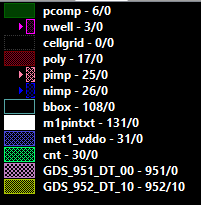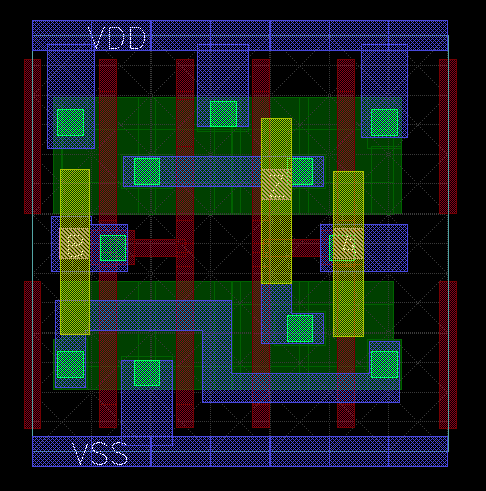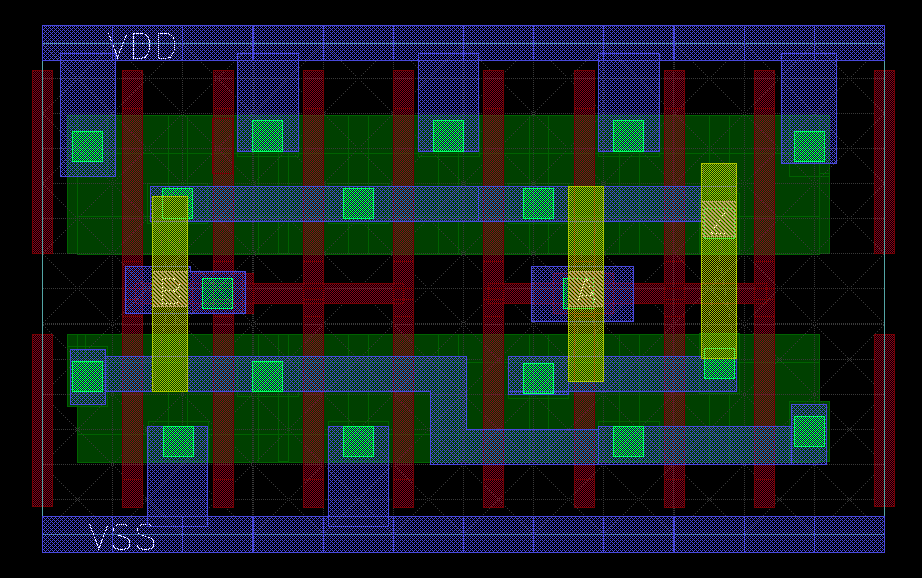# Reduce split gate option in LVS

Hi all,
I check reduction split gate option in Klayout.

Case 1: I use nand2x2 cellExtracted circuit:
M\$1 5 2 1 8 PCH L=0.04U W=0.55U AS=0.0495P AD=0.0385P PS=1.185U PD=0.83U
M\$3 5 3 1 8 PCH L=0.04U W=0.55U AS=0.04125P AD=0.05225P PS=0.85U PD=1.205U
M\$5 4 2 6 9 NCH L=0.04U W=0.255U AS=0.02535P AD=0.01785P PS=0.73U PD=0.395U
M\$6 6 2 7 9 NCH L=0.04U W=0.255U AS=0.01785P AD=0.01785P PS=0.395U PD=0.395U
M\$7 7 3 1 9 NCH L=0.04U W=0.255U AS=0.01785P AD=0.0204P PS=0.395U PD=0.415U
M\$8 1 3 4 9 NCH L=0.04U W=0.255U AS=0.0204P AD=0.02535P PS=0.415U PD=0.73U
After reduction:
M\$1 5 2 1 7 PCH L=0.04U W=0.55U AS=0.0495P AD=0.0385P PS=1.185U PD=0.83U
M\$3 5 3 1 7 PCH L=0.04U W=0.55U AS=0.04125P AD=0.05225P PS=0.85U PD=1.205U
M\$5 4 2 6 8 NCH L=0.04U W=0.51U AS=0.0432P AD=0.0357P PS=1.125U PD=0.79U
M\$7 4 3 1 8 NCH L=0.04U W=0.51U AS=0.03825P AD=0.04575P PS=0.81U PD=1.145U

this option works perfectly in this case !!!!

Case 2: I use nand2x4 cellAfter reduction:
M\$1 6 1 3 7 PCH L=0.04U W=1.1U AS=0.088P AD=0.077P PS=2.015U PD=1.66U
M\$5 6 2 3 7 PCH L=0.04U W=1.1U AS=0.077P AD=0.088P PS=1.66U PD=2.015U
M\$9 4 1 5 8 NCH L=0.04U W=0.765U AS=0.06155P AD=0.05355P PS=1.52U PD=1.185U
M\$12 5 1 9 8 NCH L=0.04U W=0.255U AS=0.01785P AD=0.01785P PS=0.395U PD=0.395U
M\$13 9 2 3 8 NCH L=0.04U W=0.255U AS=0.01785P AD=0.01785P PS=0.395U PD=0.395U
M\$14 3 2 4 8 NCH L=0.04U W=0.765U AS=0.05355P AD=0.06105P PS=1.185U PD=1.52U

But target netlist:
M\$1 6 1 4 7 PCH L=0.04U W=1.1U AS=0.088P AD=0.077P PS=2.015U PD=1.66U
M\$5 6 2 4 7 PCH L=0.04U W=1.1U AS=0.077P AD=0.088P PS=1.66U PD=2.015U
M\$9 3 1 5 8 NCH L=0.04U W=1.02U AS=0.0794P AD=0.0714P PS=1.915U PD=1.58U
M\$13 3 2 4 8 NCH L=0.04U W=1.02U AS=0.0714P AD=0.0789P PS=1.58U PD=1.915U

This option does not work perfectly in this case. It is only reduced for PMOS (PCH) and NMOS is not thorough. Can you help me fix this problem ?

Thank you very much

• edited August 2020

Hi,

The second case is lacking the symmetry requirement: there are basically two separate nodes in the low-side branch on the nand (the two serial transistors). But they are not interchangeable as one node is between two 3x transistors while the other one is between two 1x transistors.

Formally this prevents the reduction algorithm to join these nodes. There is no easy fix except for extending the algorithm. But I have no good idea how to do this currently. An option is to create a virtual connection through labels (use 'connect_implicict("nand2x4", "your_label")').

Matthias

• Thank you so much for your information

• Hi,
to resolve the issue i read netlist and join_net to joint the points like that, after that use device combine again. it work like expected. Here is my script

``````#find path
def findPath(netIgr, net, path, joined)

nnet = nil
nid = -1

net.each_terminal do |tm|
sNet = tm.device.net_for_terminal(0)
gNet = tm.device.net_for_terminal(1)
dNet = tm.device.net_for_terminal(2)
if sNet.cluster_id == netIgr.cluster_id || dNet.cluster_id == netIgr.cluster_id ;next ;end;
nnet = dNet
if nnet.cluster_id == net.cluster_id
nnet = sNet
end
if path.length == 0
path.push(nnet.cluster_id)
else
path = nnet.cluster_id
end

path.push(gNet.cluster_id)
break
end

if nnet == nil || !joined.include?(nnet.cluster_id) && nnet.terminal_count >2 || nnet.pin_count>0 ; return; end

findPath(net, nnet, path, joined)
end

def pathCompare(p1,p2)
#puts "p1_length:" + p1.length.to_s + " p2_length:" + p2.length.to_s
if p1 == nil || p2 == nil || p1.length == 0; return false; end
for i in 0..(p1.length - 1)
if p1[i] != p2[i] ; return false; end
end
return true
end

any = true
while(any)
devices.clear
any = false
top_cir.each_device do |dev|
#S-D-G-B: 0-1-2-3
if dev.device_class.name.match(reg)
next
end

s_net = dev.net_for_terminal(0)
g_net = dev.net_for_terminal(1)
d_net = dev.net_for_terminal(2)
b_net = dev.net_for_terminal(3)
if filterDevDmy
if s_net.cluster_id == d_net.cluster_id && s_net.cluster_id == g_net.cluster_id
devRemove.push(dev)
next
end
end
key_gdb = "%s_%s_%s"  %[g_net.expanded_name,d_net.expanded_name,b_net.expanded_name]
key_gsb = "%s_%s_%s"  %[g_net.expanded_name,s_net.expanded_name,b_net.expanded_name]

net_pair0 = nil
net_pair0_p = nil
i = nil
key = ""

if d_net.terminal_count != 2 && s_net.terminal_count != 2
next
end

if devices.key?(key_gdb)
i = devices[key_gdb]
key = key_gdb
net_pair0 = s_net
net_pair0_p = d_net
end

if devices.key?(key_gsb)
i = devices[key_gsb]
key = key_gsb
net_pair0 = d_net
net_pair0_p = s_net
end

if net_pair0 == nil || i == nil
if d_net.terminal_count == 2 && d_net.pin_count == 0 && d_net.pin_count == 0 && d_net.subcircuit_pin_count == 0
devices[key_gsb] = Hash.new
devices[key_gsb]["net"] = d_net
devices[key_gsb]["id"] = dev.id
end

if s_net.terminal_count == 2 && s_net.pin_count == 0 && s_net.pin_count == 0 && s_net.subcircuit_pin_count == 0
devices[key_gdb] = Hash.new
devices[key_gdb]["net"] = s_net
devices[key_gdb]["id"] = dev.id
end
next
end

net_pair = i["net"]
ddev = top_cir.device_by_id(i["id"])
net_pair_p = ddev.net_for_terminal(0)
if net_pair_p.cluster_id == net_pair.cluster_id
net_pair_p = ddev.net_for_terminal(2)
end
g1 = nil
g2 = nil
sd1 = -1
sd2 = -2
tc1 = 0
tc2 = 0
if net_pair.terminal_count ==0 || net_pair.pin_count>0 || net_pair0.pin_count>0; next; end

net_pair.each_terminal do |tm|

if tm.device.id !=i["id"] && !tm.device.device_class.name.match(reg)
g1 = tm.device.net_for_terminal(1) #net G of device
if tm.device.net_for_terminal(0).cluster_id != net_pair.cluster_id
sd1 = tm.device.net_for_terminal(0).cluster_id
tc1 = tm.device.net_for_terminal(0).terminal_count
else
sd1 = tm.device.net_for_terminal(2).cluster_id
tc1 = tm.device.net_for_terminal(2).terminal_count
end
end

end

net_pair0.each_terminal do |tm|
if tm.device.id != dev.id && !tm.device.device_class.name.match(reg)
g2 = tm.device.net_for_terminal(1) #net G of device
if tm.device.net_for_terminal(0).cluster_id != net_pair0.cluster_id
sd2 = tm.device.net_for_terminal(0).cluster_id
tc2 = tm.device.net_for_terminal(0).terminal_count
else
sd2 = tm.device.net_for_terminal(2).cluster_id
tc2 = tm.device.net_for_terminal(2).terminal_count
end
end
end

if g1 == nil || g2 == nil; next; end

if g1.cluster_id == g2.cluster_id && g1 != nil && net_pair0.cluster_id != net_pair.cluster_id && (sd1 == sd2 && tc1 !=2 || (tc1 == tc2 && tc1 == 2))
net_joined = net_joined + net_pair0.expanded_name + " vs " + net_pair.expanded_name + "\n"
pt1 = Array.new
pt2 = Array.new
findPath(net_pair0_p,net_pair0,pt1,netJoined)
findPath(net_pair_p,net_pair,pt2,netJoined)

if pathCompare(pt1, pt2)
log "Join:" + net_pair0.expanded_name + " / " + net_pair.expanded_name + " => " + pt1.to_s + " / " + pt2.to_s
top_cir.join_nets(net_pair0, net_pair)
i["net"] = net_pair0 #because net_pair merged s_net so it is not exist more
netJoined.push(net_pair0.cluster_id)
any = true
end
end

end
end
``````

dai